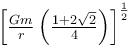Courses

# Central Forces MCQ Level – 2

## 10 Questions MCQ Test Topic wise Tests for IIT JAM Physics | Central Forces MCQ Level – 2

Description
This mock test of Central Forces MCQ Level – 2 for Physics helps you for every Physics entrance exam. This contains 10 Multiple Choice Questions for Physics Central Forces MCQ Level – 2 (mcq) to study with solutions a complete question bank. The solved questions answers in this Central Forces MCQ Level – 2 quiz give you a good mix of easy questions and tough questions. Physics students definitely take this Central Forces MCQ Level – 2 exercise for a better result in the exam. You can find other Central Forces MCQ Level – 2 extra questions, long questions & short questions for Physics on EduRev as well by searching above.
QUESTION: 1

### The gravitational potential of two homogeneous spherical shells A and B of same surface density at their respective centres are in the ratio 3 : 4. If the two shells coalesce into single one such that surface density remains same, then the ratio of potential at an internal point of the new shell of shell A is equal to :

Solution: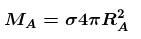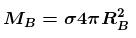where σ is surface density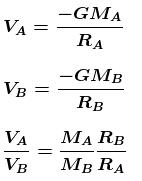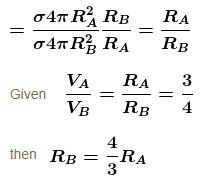for New shell of mass M and radius R.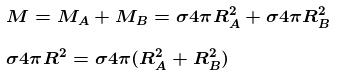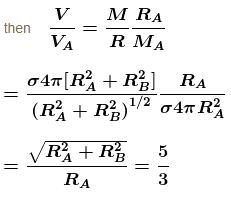The correct answer is: 5 : 3

QUESTION: 2

### A satellite is launched in the equatorial plane in such a way that it can transmit signals upto 60° latitude on the earth. The angular velocity of the satellite is :

Solution:

In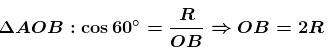(where OR is orbital radius)
Here gravitational force will provide the required centripetal force.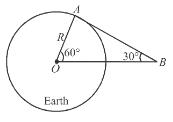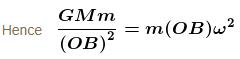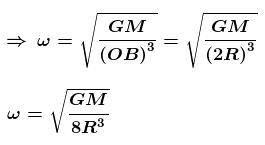The correct answer is: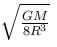QUESTION: 3

### A cavity of radius R/2 is made inside a solid sphere of radius R. The centre of the cavity is located at a distance R/2 from centre of the sphere. The gravitational force on a particle of mass m at a distance R/2 from the centre of the sphere on the line joining both the centres of sphere and cavity is (opposite to the centre of cavity). [Here,  g = GM/R2, where  M is the mass of the sphere without cavity ]

Solution:

Gravitational field at mass 'm' due to full solid sphere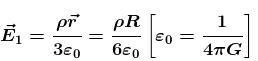Gravitational field at mass 'm' due to cavity (-ρ)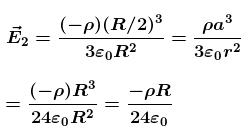Net gravitational field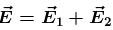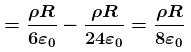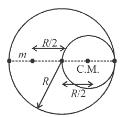Net force on m →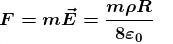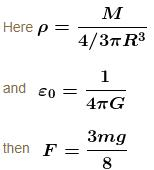QUESTION: 4

A tunnel is dug along the diameter of the earth (Radius R and mass ). There is a particle of mass m at the centre of the tunnel. The minimum velocity given to the particle so that it just reaches to the surface of the earth is :

Solution:

Let the minimum speed imparted to the particle of mass m so that it just reaches surface of earth is v. Applying conservation of energy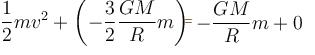Solving we get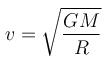The correct answer is: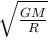QUESTION: 5

Maximum height reached by a rocket fired with a speed equal to 50% of the escape velocity from earth’s surface is :

Solution: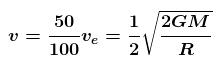Applying energy conservation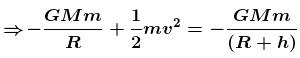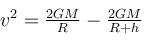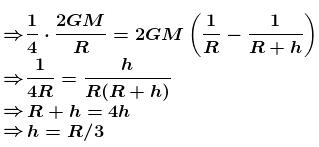QUESTION: 6

The percentage change in the acceleration of the earth towards the sun from a total eclipse of the sun to the point where the moon is on a side of earth directly opposite to the sun is :

Solution:

During total eclipse-
Total attraction due to sun and moon.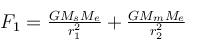When moon goes on the opposite side of earth.
Effective force of attraction,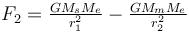Change in force,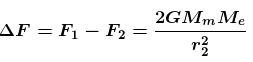Change in acceleration of earth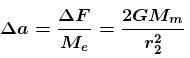Average force on earth,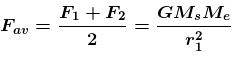Average acceleration of earth,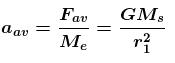%age change in acceleration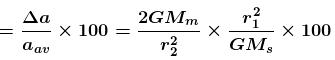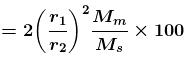The correct answer is: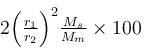QUESTION: 7

A small area is removed from a uniform spherical shell of mass M and radius R. Then the gravitational field intensity near the hollow portion is :

Solution:

The Gravitational field at the centre of the hole due to the remaining mass is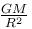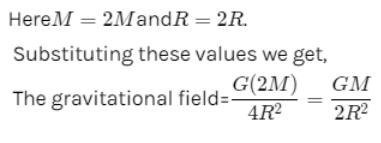QUESTION: 8

A point P lies on the axis of a fixed ring of mass M and radius R, at a distance 2R from its centre O. A small particle starts from P and reaches O under gravitational attraction only. Its speed O will be

Solution:

Gravitational potential at ‘P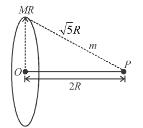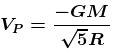Gravitational potential at ‘O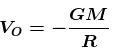work energy theorem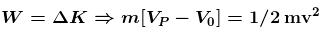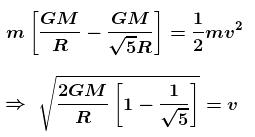The correct answer is: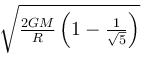QUESTION: 9

Two particles of combined mass M, placed in space with certain separation, are released. Interaction between the particles is only of gravitational nature and there is no external force present. Acceleration of one particle with respect to the other when separation between them is R, has a magnitude :

Solution: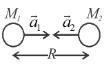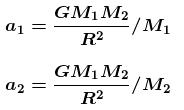acceleration of M1 w.r.t. M2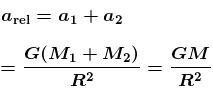The correct answer is: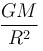QUESTION: 10

Four similar particles of mass m are orbiting in a circle of radius r in the same angular direction because of their mutual gravitational attractive force. Velocity of a particle is given by :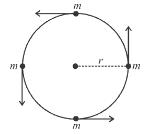Solution:

Centripetal force = net gravitational force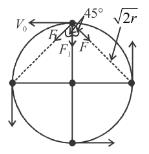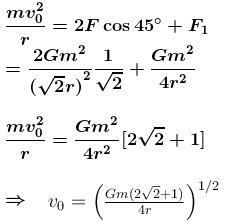The correct answer is: